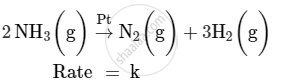Department of Pre-University Education, KarnatakaPUC Karnataka Science Class 12

# For a reaction (i) Write the order and molecularity of this reaction. (ii) Write the unit of k. - Chemistry

For a reaction :(i) Write the order and molecularity of this reaction.

(ii) Write the unit of k.

#### Solution

(i) This reaction is catalysed by Pt at high pressure. So, it is a zero-order reaction with molecularity 2.

(ii) The rate law expression for this reaction is

Rate = k

Hence, the unit of k is mol L−1 s−1.

Concept: Factors Influencing Rate of a Reaction
Is there an error in this question or solution?